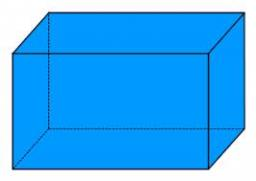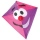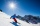# Cuboid

Cuboid with edge a=6 cm and body diagonal u=31 cm has volume V=900 cm3. Calculate the length of the other edges.

Result

b =  30 cm
c =  5 cm

#### Solution:

Checkout calculation with our calculator of quadratic equations.

$c=c_{2}=5 \ \text{cm} \ \\ \ \\ \text{ Correctness test: } \ \\ d=\sqrt{ a^2+b^2+c^2 }=\sqrt{ 6^2+30^2+5^2 }=31 \ \\ d=u \ \\ V_{2}=a \cdot \ b \cdot \ c=6 \cdot \ 30 \cdot \ 5=900 \ \text{cm}^3 \ \\ V_{2}=V$Our examples were largely sent or created by pupils and students themselves. Therefore, we would be pleased if you could send us any errors you found, spelling mistakes, or rephasing the example. Thank you!

Leave us a comment of this math problem and its solution (i.e. if it is still somewhat unclear...):Be the first to comment!Tips to related online calculators
Looking for help with calculating roots of a quadratic equation?
Do you have a system of equations and looking for calculator system of linear equations?
Do you want to convert length units?
Tip: Our volume units converter will help you with the conversion of volume units.
Pythagorean theorem is the base for the right triangle calculator.

## Next similar math problems:

1. Height of the cuboidCuboid with a rectangular base, measuring 3 cm and 4 cm diagonal has a body 13 centimeters long. What is the height of the cuboid?
2. Wall and body diagonalsThe block/cuboid has dimensions a = 4cm, b = 3cm and c = 12cm. Calculates the length of the wall and body diagonals.
3. EmbankmentThe railway embankment 300 m long has a cross section of an isosceles trapezoid with bases of 14 m and 8 m. The trapezoidal arms are 5 m long. Calculate how much m3 of soil is in the embankment?
4. Concentric circles and chordIn a circle with a diameter d = 10 cm, a chord with a length of 6 cm is constructed. What radius have the concentric circle while touch this chord?
5. The basesThe bases of the isosceles trapezoid ABCD have lengths of 10 cm and 6 cm. Its arms form an angle α = 50˚ with a longer base. Calculate the circumference and content of the ABCD trapezoid.
6. Annulus from triangleCalculate the content of the area bounded by a circle circumscribed and a circle inscribed by a triangle with sides a = 25mm, b = 29mm, c = 36mm
7. Cone roofHow many m2 of roofing is needed to cover a cone-shaped roof with a diameter of 10 m and a height of 4 m? Add an extra 4% to the overlays.
8. An equilateralAn equilateral triangle is inscribed in a square of side 1 unit long so that it has one common vertex with the square. What is the area of the inscribed triangle?
9. ProbabilityHow probable is a randomly selected three-digit number divisible by five or seven?
10. The right triangleThe right triangle ABC has a leg a = 36 cm and an area S = 540 cm2. Calculate the length of the leg b and the median t2 to side b.
11. FlakesA circle was described on the square, and a semicircle above each side of the square was described. This created 4 "flakes". Which is bigger: the content of the central square or the content of four chips?
12. Spherical capThe spherical cap has a base radius of 8 cm and a height of 5 cm. Calculate the radius of a sphere of which this spherical cap is cut.
13. The rescue helicopterThe rescue helicopter is above the landing site at a height of 180m. The site of the rescue operation can be seen from here at a depth angle of 52° 40 '. How far will the helicopter land from the rescue site?
14. A kiteChildren have a kite on an 80m long rope, which floats above a place 25m from the place where children stand. How high is the dragon floating above the terrain?
15. RT triangle and heightCalculate the remaining sides of the right triangle if we know side b = 4 cm long and height to side c h = 2.4 cm.
16. Black diamond runTaleah is skiing down a black diamond run. She begins skiing at the top of a ski trail whose elevation is about 8625 feet. The ski run ends toward the base of the mountain at 3800 feet. The horizontal distance between these two points is about 4775 feet.
17. The angle of viewDetermine the angle of view at which the observer sees a rod 16 m long when it is 18 m from one end and 27 m from the other.